# Access, rank and select queries on bitmaps

## What is access?

Access tells you the value at a given index in a bitmap.

### Example 1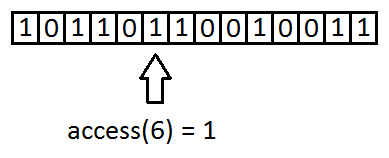### Example 2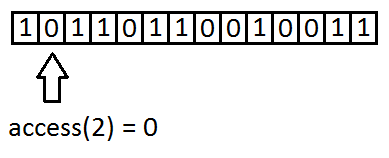In programming sense it is a function with 2 arguments:

```int access(void *bitmap, int index)
{
// ...
return value;
}```

## What is rank?

Rank tells you how many 1s are in a bitmap till a given index.

### Example 1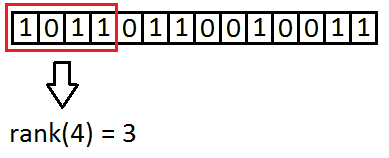### Example 2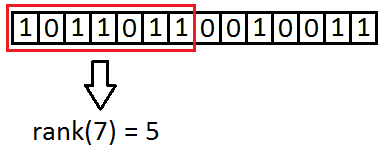In programming sense it is a function with 2 arguments:

```int rank(void *bitmap, int index)
{
// ...
return numofones;
}```

## What is select?

Select tells you at which index is the n-th 1 in a bitmap.

### Example 1### Example 2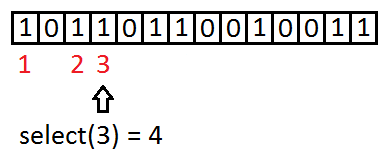In programming sense it is a function with 2 arguments:

```int select(void *bitmap, int numofones)
{
// ...
return index;
}```

## What is the use of these queries?

They are used in implementations of succinct data structures. With these structures data can be compressed in a way to be able to use in-place, without decompressing it first. By using these queries on these structures you can solve a lot of problems.

In these examples bitmap should be compressed of course by using a succinct data structure (like RRR) for size and an efficient rank/select implementation.

### Example 1

You have a text which consists of words.

1. You want to get the nth word of this text.
2. You want to know how many words are in a range.

How can you accomplish this?

Make a bitmap which maps the words’ start position.
In this bitmap:
– 1 means a word’s start
– 0 means other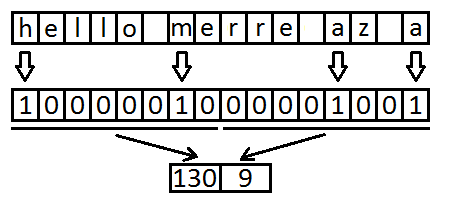With select you can solve the first problem:

`index = select(bitmap, n);`

Now you can read the nth word from this index.

With rank you can determine the words number in a range (a,b):

`numofwords = rank(bitmap, b) - rank(bitmap, a);`

Notice:
There should be a check here for the last word’s end (it can be out of range).

### Example 2

There is a long line of containers at customs authority. Some are empty, others are filled with goods.

There are not enough officiers to check for all the containers (verifing if it contains what is written on it).
Check every nth occupied container!

You have a bitmap which maps containers.
In this bitmap:
– 1 means a container is filled with goods
– 0 means a container is empty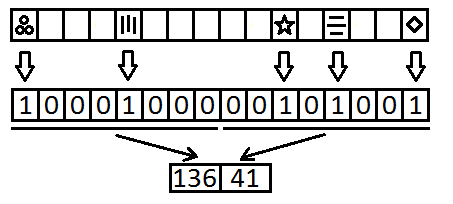With select you can solve this problem:

```index1 = select(n);
index2 = select(2*n);
// ..```

### Example 3

There is a large prison with lot of cells. A cell is empty or occupied by one prisoner. The cells are indexed in lines. The security system failed on one passage and all cells on this line accidentally opened.

How many prisoners did escape?

You have a bitmap which maps cells.
In this bitmap:
– 1 means a cell is occupied by a prisoner
– 0 means a cell is empty

With rank you can solve the problem:

`number = rank(index_end) - rank(index_start);`

This site uses Akismet to reduce spam. Learn how your comment data is processed.